Latest Banking jobs   »   Reasoning Ability Quiz For IBPS Clerk...

# Reasoning Ability Quiz For IBPS Clerk Prelims 2021- 4th December

Directions (1-5): Study the information carefully and answer the questions given below.
Eight persons P, Q, R, S, T, U, V and W are going for holidays on two different dates (13th and 22nd) in four different months i.e. January, March, April and May. All information is not necessarily in same order.
Q goes in April. Three person goes between Q and T. Equal number of persons goes after T and Before R. More than one person goes between R and S who does not goes on odd number date. One person goes between P and W. Equal number of person goes after V and Before U who goes on odd number date. P does not go in the month of Q.

Q1. Who among the following person goes on 13th January?
(a) V
(b) T
(c) S
(d) Q
(e) None of these

Q2. How many persons goes between V and S?
(a) One
(b) Three
(c) Four
(d) Five
(e) None of these

Q3. Who among the following person goes on even number date of the month which has even number of days?
(a) W
(b) Q
(c) U
(d) V
(e) None of these

Q4. How many persons go before S?
(a) Two
(b) One
(c) Four
(d) Five
(e) None of these

Q5. Which of the following combination is true?
(a)13th January-Q
(b) 22nd April-R
(c) 13th April-V
(d) 22nd March-P
(e) None is true

Directions (6-8): Study the given information and answer the following question.
Eight pillars are arranged in a ground in a certain way. A is 20m east of B. G is 15m west of D. H is 5m east of C which is 5m north of F. E is 16m south of G. D is 8m north of A. E is 10m east of F.

Q6. What is the shortest distance between A and E?
(a) 18m
(b) 15m
(c) 17m
(d) 21m
(e) 22m

Q7. Pillar A is in which direction with respect to H?
(a) North-East
(b) South-East
(c) East
(d) North-West
(e) South-West

Q8. What is the shortest distance between Pillar B and Pillar C?
(a) 6m
(b) 5m
(c) √35m
(d) 37m
(e) None of these

Directions (9-10): Study the following information carefully and answer the question given below-
There are eight members in a family i.e. K, O, L, N, Q, M, P and S. Among them three are married couples. S is the daughter of the one who is L’s nephew. Q is the daughter-in-law of the one who is grandfather of M. O has only one grandson. P is grand-daughter-in-law of K. L is O’s husband’s sister.

Q9.How many children P’s father-in-law has?
(a) Two
(b) One
(c) Three
(d) Four
(e) None of these

Q10. Who among the following is father of N?
(a) O
(b) L
(c) K
(d) M
(e) None of these

Directions (11-12): In each of the questions below. Some statements are given followed by conclusions/group of conclusions numbered I and II. You have to assume all the statements to be true even if they seem to be at variance from the commonly known facts and then decide which of the given two conclusions logically follows from the information given in the statements.
(a) If only conclusion I follows
(b) If only conclusion II follows
(c) If either I or II follows
(d) If neither I nor II follows
(e) If both I and II follow

Q11. Statements:
No Glitter is Bracelet
All Locket are Bracelet
Some locket are Ring
Conclusions:
I: Some Ring are not glitter
II: No locket is glitter

Q12. Statements:
All Ball are Cat
Only a few Ball are Apple
Some Eagle are Cat
Conclusions:
I: Some Cat are Apple
II: All Eagle are Ball is a possibility

Directions (13-15): Study the following information carefully and answer the given questions. In a certain code language,
‘Temperature sun summer rise’ is coded as ‘% @ # \$’
‘Sun Pollution seasonal summer’ is coded as ‘# \$ ~ >’
‘Temperature summer warmer Pollution’ is coded as ‘? ~ # @’

Q13. What may be the code of ‘World Temperature’?
(a) @ #
(b) @ >
(c) @ ?
(d) @ *
(e) @ ~

Q14. Which of the following code is coded for ‘Pollution’?
(a) @
(b) ~
(c) \$
(d) ?
(e) None of these

Q15. What will be the code of ‘seasonal green’?
(a) > ?
(b) ? @
(c) > \$
(d) @ >
(e) None of these

Solutions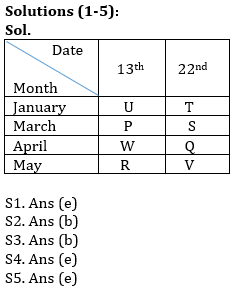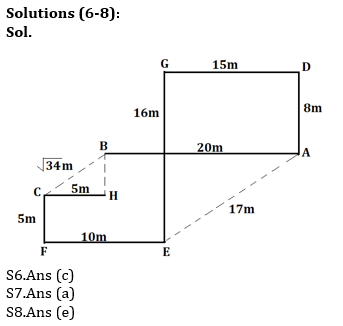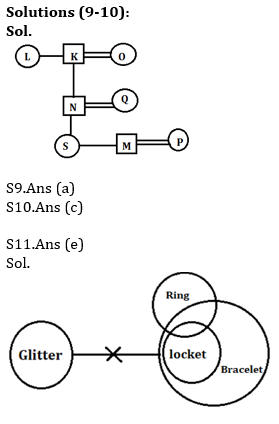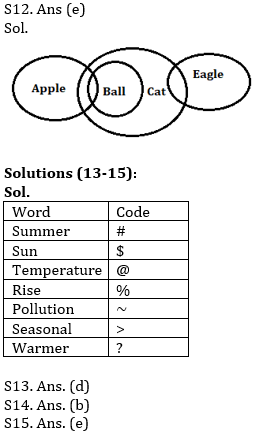#### Congratulations!Incorrect details? Fill the form again here

•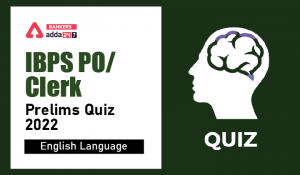English Quizzes For IBPS Clerk/PO Prelim...
•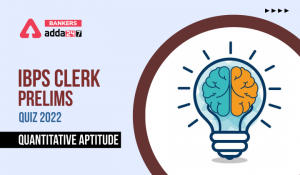Quantitative Aptitude Quiz For IBPS Cler...
•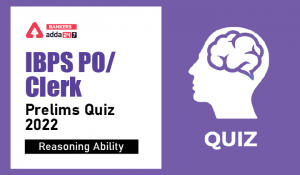Reasoning Ability Quiz For IBPS Clerk/PO...
•Quantitative Aptitude Quiz For IBPS Cler...
•Reasoning Ability Quiz For IBPS RRB PO C...
•Reasoning Ability Quiz For IBPS Clerk/PO...